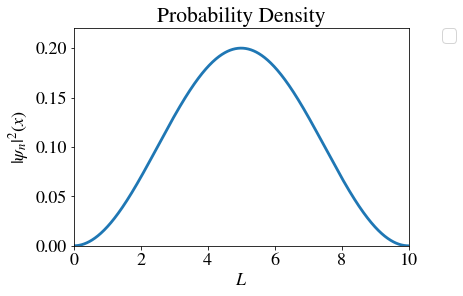# 2.3: The Schrödinger Equation for a Wave

•• Valeria D. Kleiman
• University of Florida
$$\newcommand{\vecs}{\overset { \rightharpoonup} {\mathbf{#1}} }$$ $$\newcommand{\vecd}{\overset{-\!-\!\rightharpoonup}{\vphantom{a}\smash {#1}}}$$$$\newcommand{\id}{\mathrm{id}}$$ $$\newcommand{\Span}{\mathrm{span}}$$ $$\newcommand{\kernel}{\mathrm{null}\,}$$ $$\newcommand{\range}{\mathrm{range}\,}$$ $$\newcommand{\RealPart}{\mathrm{Re}}$$ $$\newcommand{\ImaginaryPart}{\mathrm{Im}}$$ $$\newcommand{\Argument}{\mathrm{Arg}}$$ $$\newcommand{\norm}{\| #1 \|}$$ $$\newcommand{\inner}{\langle #1, #2 \rangle}$$ $$\newcommand{\Span}{\mathrm{span}}$$ $$\newcommand{\id}{\mathrm{id}}$$ $$\newcommand{\Span}{\mathrm{span}}$$ $$\newcommand{\kernel}{\mathrm{null}\,}$$ $$\newcommand{\range}{\mathrm{range}\,}$$ $$\newcommand{\RealPart}{\mathrm{Re}}$$ $$\newcommand{\ImaginaryPart}{\mathrm{Im}}$$ $$\newcommand{\Argument}{\mathrm{Arg}}$$ $$\newcommand{\norm}{\| #1 \|}$$ $$\newcommand{\inner}{\langle #1, #2 \rangle}$$ $$\newcommand{\Span}{\mathrm{span}}$$$$\newcommand{\AA}{\unicode[.8,0]{x212B}}$$

## The time-independent Schrödinger equation

When matter is described as waves, we can use a wave-equation to describe it mathematically. The wave equation will describe how the wave evolves in time and in space. In here, we will focus on the properties of the wave in space, thus the governing equation for a wave-particle in a one dimensional space (first proposed by Edwin Schrödinger in 1926) is

$\textcolor{purple}{-\dfrac{\hbar}{2m} \ \dfrac{d^2\textcolor{black}{\psi (x)}}{dx^2}} + \textcolor{purple}{V(x)}\ \psi(x)= \textcolor{darkgreen}{E} \cdot\psi(x)$

Caution

Don't panic. We want to understand the equation but we will not use its math

There are 3 components to this equation

• $$\psi(x)$$ is the function of the wave that describes the particle (wavefunction). If we know how to express $$\psi(x)$$ and how to evaluate it for values of x, then we can evaluate all the properties of the particle-wave that can be measured in a laboratory. For a given particle-wave, a specific wavefunction $$\psi(x)$$ is called a state. For an atom or molecule, each $$\psi(x)$$ is called an orbital.
• $$\textcolor{darkgreen}{E}$$ is the numerical value (in units of energy) that corresponds to the energy of the particle-wave in the specific state represented by $$\psi(x)$$.
• $$\textcolor{purple}{-\dfrac{\hbar}{2m}\dfrac{d^2}{dx^2}}$$ and$$\textcolor{purple}{V(x)}$$ are called operators. The sum$$\textcolor{purple}{-\dfrac{\hbar}{2m}\dfrac{d^2}{dx^2} +V(x)=H}$$ is called the Hamiltonian operator.

Example $$\PageIndex{1}$$: The wavefunction of a particle in a box

Consider an electron that can move in one-dimensional space (moving within a line). If this electron is confined within a 1-D box of length 10 Å its state will be described by $\psi_1(x) = \sqrt{\dfrac{2}{10 Å}} sin( \dfrac{ \pi}{10 Å} \cdot x )$

Solution

Since we know the wavefunction for the particular state 1, we can plot the probability density for all possible positions in the box where the particle could be found.

$|\psi_1(x)|^2 = \dfrac{2}{10 Å} \left(sin ( \dfrac{ \pi}{10 Å} \cdot x )\right)^2$If we were to measure in the lab the position of this particle, there would be a 0.07 probability to find it at $$x=8 Å$$. The energy of state 1 is given by $$\textcolor{darkgreen}{E = 6.025 \cdot 10^{-20} Joules }$$

To describe particle-waves that can move in 3 dimensions (for example, the H atom, with 1 electron and 1 proton), we use a 3-dimensional wavefunction $$\psi(x,y,z)$$ with the Schrödinger equation also written for 3 dimensions. The probability density would be plotted in 4 dimensions (one for each coordinate $$x, y, z$$, and a 4th for the value of Probability Density at each point in space $$|\psi (x,y,z)|^2$$.

This page titled 2.3: The Schrödinger Equation for a Wave is shared under a not declared license and was authored, remixed, and/or curated by Valeria D. Kleiman.